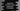# TypeScript for and for-in loop explanation & examples# Introduction :

Typescript for loop and for-in loops are used to execute a piece of code repeatedly. It checks one condition and if the condition is true, it executes. It runs the code piece repeatedly until the execution condition is true. Once it becomes false, it stops, quits the loop and executes the next steps of the program. In this tutorial, we will learn two different variants of the for loop in typescript: original for loop and for in loop.

## Syntax of for loop :

The syntax of for loop is as below :

``````for(variable_initial_value; condition; variable_update_condition){
//code block
}``````

Here, variableinitialvalue : It is used to initialize the variable with a value. condition : This is the condition of the for loop i.e. the for loop will run till the condition is true. variableupdatecondition : This condition is used to update the variable at the end of the execution of each iteration of the loop.

### Example of for loop :

One example of the typescript for loop is as below :

``````for(var i:number = 1; i<5; i++){
console.log(`Execution step : \${i}`)
}``````

It will print the below output :

``````Execution step : 1
Execution step : 2
Execution step : 3
Execution step : 4``````

### Explanation :

In this example, number i is used as the for loop variable. Its initial value is 1 and it increments by 1 at the end of each iteration. Also, the loop runs till i is less than 5. As you have seen in the output, the loop executed for 4 times with different values of i on each execution.

## for…in loop :

Typescript provides one different form of the for loop called for…in loop. This loop is used to iterate through a list of collection like array,tuple, list etc. Using this loop, we can easily iterate through such collection. The syntax of for…in loop is as below :

``````for(var i in data){
//code block
}``````

Here, i is the current index.

### Example of for…in loop :

We can iterate through an array of numbers using for loop as like below :

``````var numArr:number[] = [1,2,3,4,5];

for(var i = 0; i<numArr.length; i++){
console.log(`value for index \${i} : \${numArr[i]}`);
}``````

It will print the below output :

``````value for index 0 : 1
value for index 1 : 2
value for index 2 : 3
value for index 3 : 4
value for index 4 : 5``````

Using for…in loop, we can write the same program as like below :

``````var numArr:number[] = [1,2,3,4,5];

for(var i in numArr){
console.log(`value for index \${i} : \${numArr[i]}`);
}``````

It prints the same output.

for…in loop is really useful for data set like an array, tuple, etc.

### Conclusion :

Like any other programming language, for loop is an integral part of typescript. We have learned two different types of for loop in this program. Try to go through the examples explained above and drop one comment below if you have any queries.